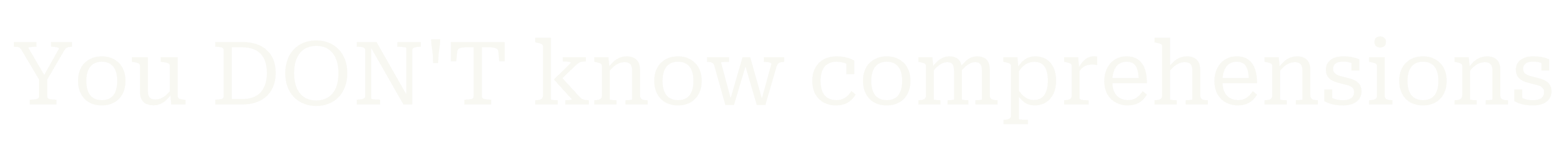``````squares = []
for num in range(10):
squares.append(num ** 2)``````
``````my_list = []
[my_list.append(num ** 2) for num in range(10)]``````
``[print(num ** 2) for num in range(10)]``
``````[func(value) for value in data_source)]
[value for value in data_source if predicate(value)``````
``my_list = [value for value in iterable]``
``colour = [randint(0, 255) for _ in range(3)]``
``my_list = [value for value, key in zip(values, keys) if predicate(keys)]``
``````my_list = [
transformation(elem)
for elem in data_source
if predicate(elem)
]``````
``````squares = []
for num in range(10):
squares.append(num ** 2)``````
``squares = [num ** 2 for num in range(10)]``

Equivalent to a for loop with an append.

Equivalent to a for loop with an if and an append.

You don't know comprehensions.

It's ok!

Convenient syntax to create lists.

Builds a new list out of an existing iterable.

The list comprehension extracts important bits...

• Shorter

• Faster (objectively)

2 common patterns.

• Own scope (pure)

• IT EMPHASISES AND HIGHLIGHTS

THE CONTENTS OF THE NEW LIST.

... and drops fluff:

What is a list comprehension?

Filtering

Anatomy

More examples

Tips

Do you know list comprehensions?

Yes

What's the

of using them?

They are fast.

They are short.

Wait!

What was that?

???

I don't know 🤷

No

``````[
0,  1,  4,  9, 16,
25, 36, 49, 64, 81,
]``````
``````[
1,      9,
25,     49,     81,
]``````
``squares = [num ** 2 for num in range(10)]``
``````squares = []
for num in range(10):
squares.append(num ** 2)``````
``squares = [num ** 2 for num in range(10) if num % 2]``
``````american_actors = [
actor
for actor, nationality in actors.items()
if nationality == "USA"
]``````
``````e_word_lengths = [
len(word)
for word in sentence.split()
if "e" in word
] # == ???``````
``````e_word_lengths = []
for word in sentence.split():
if "e" in word:
e_word_lengths.append(len(word))
print(word)``````
``````squares = [
num ** 2
for num in range(10)
if num % 2
]``````
``````squares = [
num ** 2
for num in range(10)
if num % 2
]``````
``````new_list = [
func(elem)
for elem in data_source
if predicate(elem)
]``````
``````new_list = [
func(elem)
for elem in data_source
if predicate(elem)
]``````
``````
func = elem ** 2
data_source = range(10)
predicate = elem % 2
``````
``````
func = len(word)
data_source = sentence.split()
predicate = "e" in word
``````
``````squares = []
for num in range(10):
if num % 2:
squares.append(num ** 2)``````

1. a transformation

applied to the existing data

2. a data source

to fetch the elements from

In general, list comprehensions have 3 components:

Converting to/from a list comprehension is “straightforward”.

The only thing that moves is the transformation.

General form

Specific example

3. an optional filter

to select which elements to use

``````new_list = [
func(elem)
for elem in data_source
if predicate(elem)
]``````
``````new_list = []
for elem in data_source:
if predicate(elem):
new_list.append(func(elem))``````

1. transformation

The data transformation comes first in the comp. but last in the loop.

The loop is just a comprehension with extra steps.

• Unused list comp?

• Don't reinvent map

& filter!!!